JAJSE80R October   2017  – November 2021

PRODUCTION DATA

1. 特長
2. アプリケーション
3. 説明
4. Revision History
5. Device Comparison Table
6. Pin Configuration and Functions
7. Specifications
8. Detailed Description
9. Application and Implementation
1. 9.1 Application Information
2. 9.2 Typical Application
1. 9.2.1 TLV900x Low-Side, Current Sensing Application
2. 9.2.2 Single-Supply Photodiode Amplifier
10. 10Power Supply Recommendations
11. 11Layout
12. 12Device and Documentation Support
13. 13Mechanical, Packaging, and Orderable Information

#### パッケージ・オプション

デバイスごとのパッケージ図は、PDF版データシートをご参照ください。

• RUC|14
• D|14
• DYY|14
• PW|14
• RTE|16
• RTE|16
• PW|14
• D|14

## 7.11 Typical Characteristics

at TA = 25°C, V+ = 2.75 V, V– = –2.75 V, RL = 10 kΩ connected to VS / 2, VCM = VS / 2, and VOUT = VS / 2 (unless otherwise noted)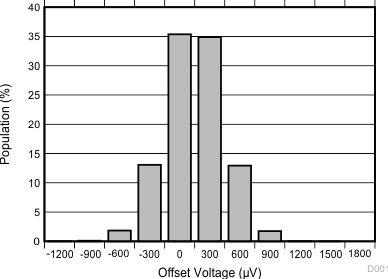VS = 5 V
Figure 7-1 Offset Voltage Distribution Histogram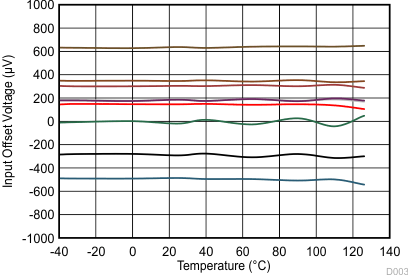Figure 7-3 Input Offset Voltage vs Temperature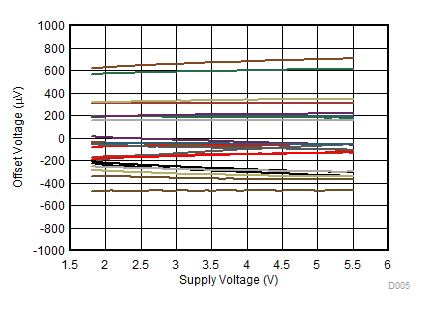Figure 7-5 Offset Voltage vs Supply Voltage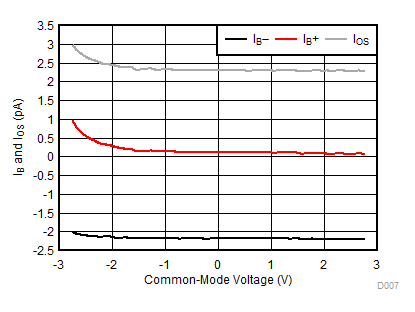Figure 7-7 IB and IOS vs Common-Mode Voltage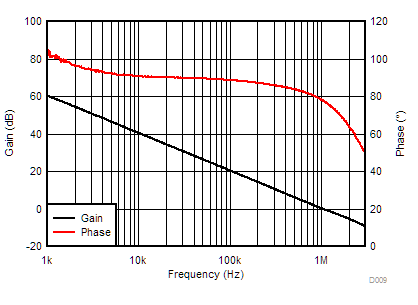CL = 10 pF
Figure 7-9 Open-Loop Gain and Phase vs Frequency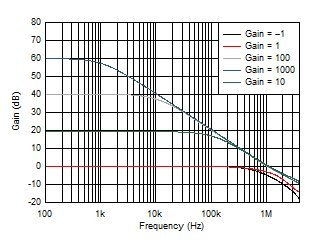CL = 10 pF
Figure 7-11 Closed-Loop Gain vs Frequency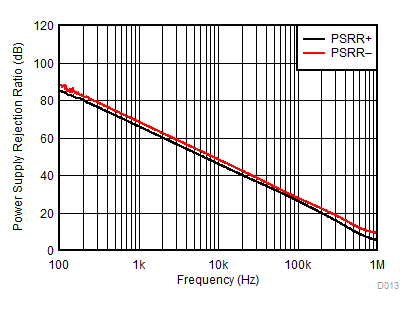Figure 7-13 PSRR vs Frequency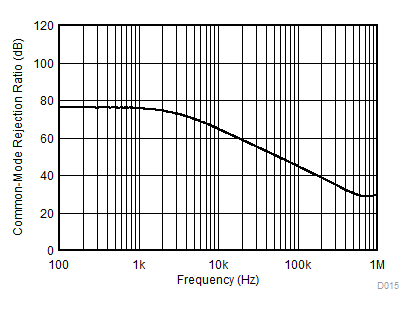Figure 7-15 CMRR vs Frequency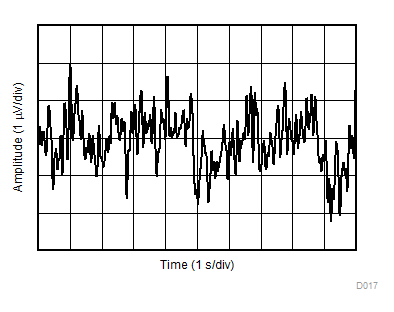Figure 7-17 0.1-Hz to 10-Hz Integrated Voltage Noise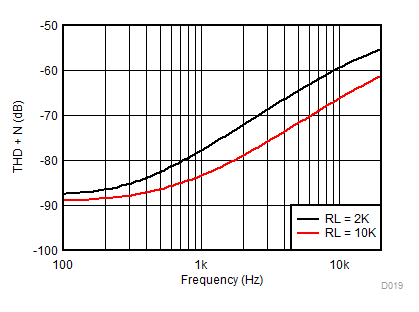VS = 5.5 V VCM = 2.5 V G = 1 BW = 80 kHz VOUT = 0.5 VRMS
Figure 7-19 THD + N vs Frequency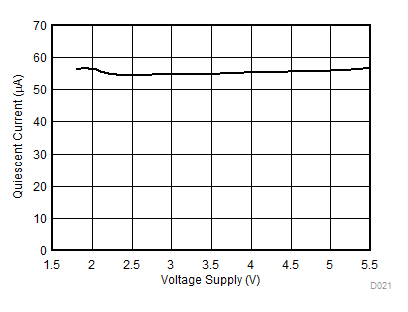Figure 7-21 Quiescent Current vs Supply Voltage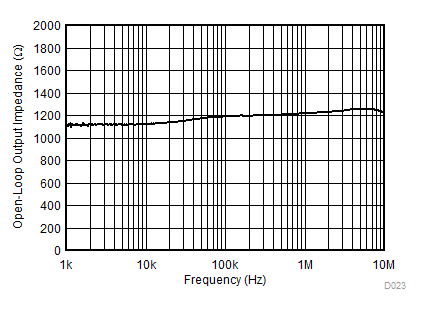Figure 7-23 Open-Loop Output Impedance vs Frequency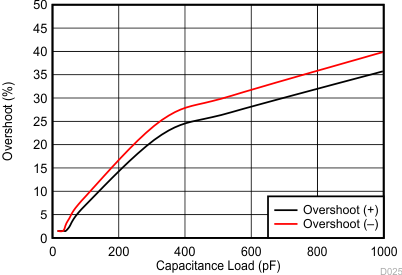G = –1 VIN = 100 mVpp
Figure 7-25 Small Signal Overshoot vs Capacitive Load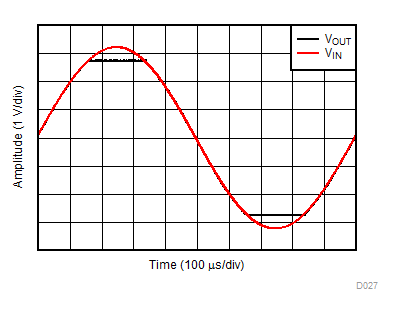G = 1 VIN = 6.5 VPP
Figure 7-27 No Phase ReversalG = 1 VIN = 100 mVPP CL = 10 pF
Figure 7-29 Small-Signal Step Response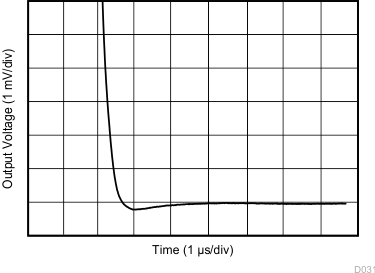G = 1 CL = 100 pF 2-V step
Figure 7-31 Large-Signal Settling Time (Negative)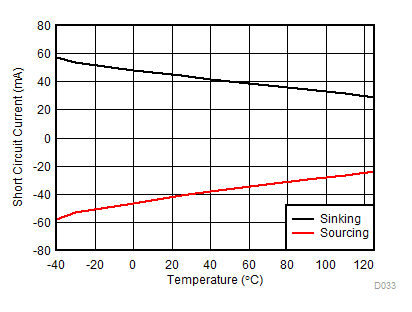Figure 7-33 Short-Circuit Current vs Temperature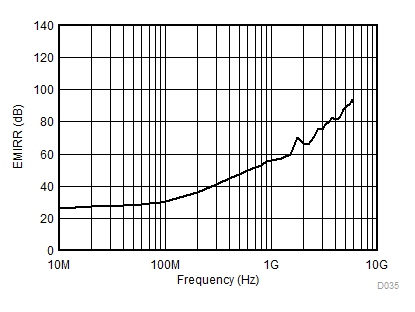Figure 7-35 Electromagnetic Interference Rejection Ratio Referred to Noninverting Input (EMIRR+) vs FrequencyVS = 5 V, TA = –40°C to 125°C
Figure 7-2 Offset Voltage Drift Distribution Histogram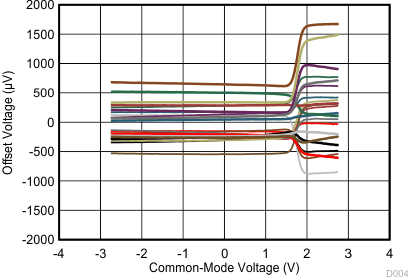Figure 7-4 Offset Voltage vs Common-Mode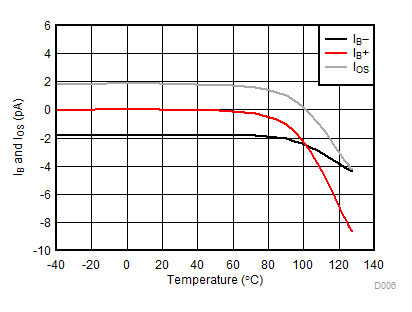Figure 7-6 IB and IOS vs Temperature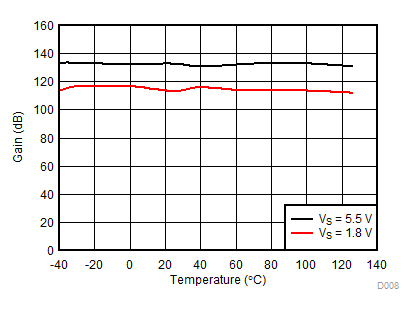Figure 7-8 Open-Loop Gain vs Temperature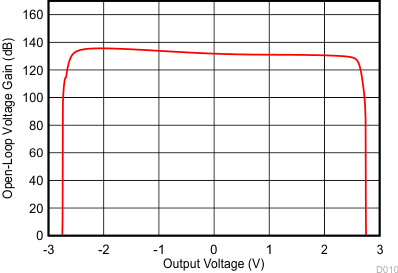Figure 7-10 Open-Loop Gain vs Output Voltage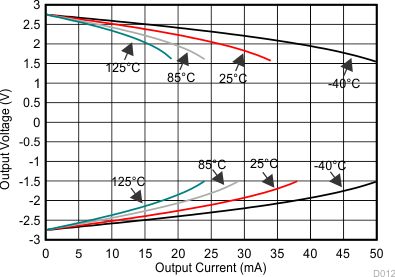Figure 7-12 Output Voltage vs Output Current (Claw)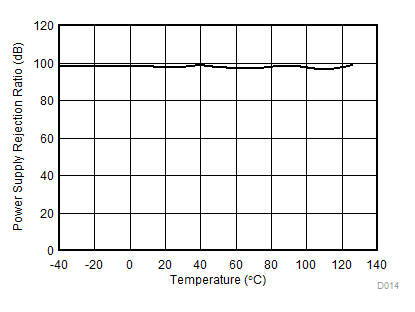VS = 1.8 V to 5.5 V
Figure 7-14 DC PSRR vs Temperature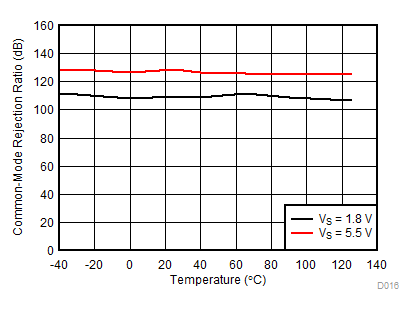VCM = (V–) – 0.1 V to (V+) – 1.4 V
Figure 7-16 DC CMRR vs Temperature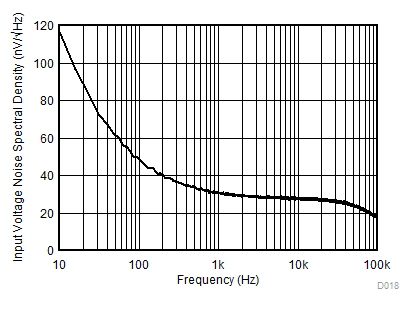Figure 7-18 Input Voltage Noise Spectral Density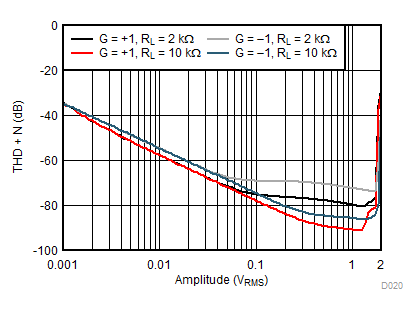VS = 5.5 V VCM = 2.5 V ƒ = 1 kHz G = 1 BW = 80 kHz
Figure 7-20 THD + N vs Amplitude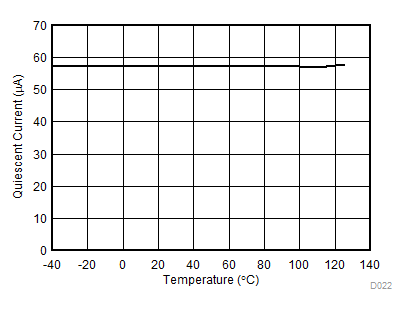Figure 7-22 Quiescent Current vs Temperature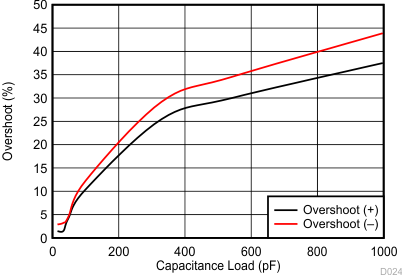G = 1 VIN = 100 mVpp
Figure 7-24 Small Signal Overshoot vs Capacitive LoadFigure 7-26 Phase Margin vs Capacitive Load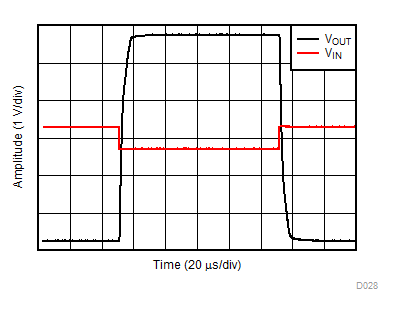G = –10 VIN = 600 mVPP
Figure 7-28 Overload Recovery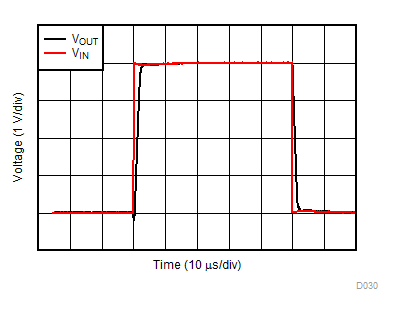G = 1 VIN = 4 VPP CL = 10 pF
Figure 7-30 Large-Signal Step Response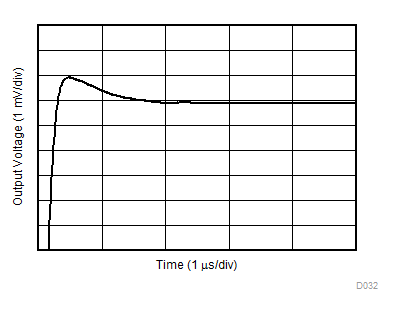G = 1 CL = 100 pF 2-V step
Figure 7-32 Large-Signal Settling Time (Positive)Figure 7-34 Maximum Output Voltage vs FrequencyFigure 7-36 Channel Separation